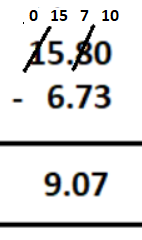Question

is equal to

A
B
C
D
Medium

SolutionVerified by Toppr
Correct option is B)

To subtract two decimals, convert 15.8 into like decimals with 2 decimal places,Now, subtracting decimals we get,00